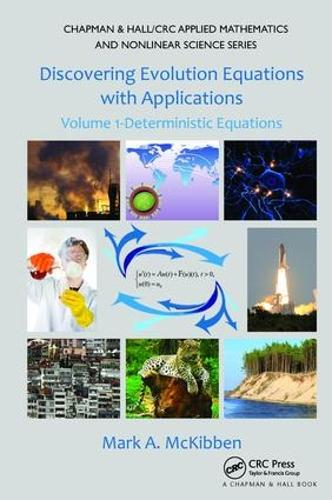•# Discovering Evolution Equations with Applications: Volume 1-Deterministic Equations - Chapman & Hall/CRC Applied Mathematics & Nonlinear Science (Paperback)

(author)
£61.99
Paperback 466 Pages / Published: 14/06/2017
• We can order this

Usually dispatched within 3 weeks

Discovering Evolution Equations with Applications: Volume 1-Deterministic Equations provides an engaging, accessible account of core theoretical results of evolution equations in a way that gradually builds intuition and culminates in exploring active research. It gives nonspecialists, even those with minimal prior exposure to analysis, the foundation to understand what evolution equations are and how to work with them in various areas of practice.

After presenting the essentials of analysis, the book discusses homogenous finite-dimensional ordinary differential equations. Subsequent chapters then focus on linear homogenous abstract, nonhomogenous linear, semi-linear, functional, Sobolev-type, neutral, delay, and nonlinear evolution equations. The final two chapters explore research topics, including nonlocal evolution equations. For each class of equations, the author develops a core of theoretical results concerning the existence and uniqueness of solutions under various growth and compactness assumptions, continuous dependence upon initial data and parameters, convergence results regarding the initial data, and elementary stability results.

By taking an applications-oriented approach, this self-contained, conversational-style book motivates readers to fully grasp the mathematical details of studying evolution equations. It prepares newcomers to successfully navigate further research in the field.

Publisher: Taylor & Francis Ltd
ISBN: 9781138117785
Number of pages: 466
Weight: 454 g
Dimensions: 235 x 159 mm